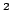Statistica Sinica 20 (2010), 637-659

SIMULTANEOUS CONFIDENCE INTERVALS FOR

SEMIPARAMETRIC LOGISTICS REGRESSION

AND CONFIDENCE REGIONS FOR

THE MULTI-DIMENSIONAL EFFECTIVE DOSE

Jialiang Li, Chunming Zhang, Kjell A. Doksumand Erik V. NordheimNational University of Singapore andUniversity of Wisconsin, Madison

Abstract: We construct pointwise and simultaneous confidence intervals for the link function in a semiparametric logistic regression model based on the logit link function. Simultaneous confidence intervals are especially important for semiparametirc regression models since they allow inference to be made over the whole predictor space. These intervals are used to construct confidence regions for the multi-dimensional effective dose - that is, for the set of multivariate covariate values where the probability that the binary response is one is equal to a preassigned value. We construct methods using inequalities, asymptotics and a semiparametric bootstrap. Simulations and examples are provided to demonstrate the performance and to compare the methods. The bootstrap procedure yields accurate confidence regions for the multi-dimensional effective dose.

Key words and phrases: Bootstrap, local likelihood, multi-dimensional effective dose, semiparametric logistic regression model, simultaneous confidence interval.